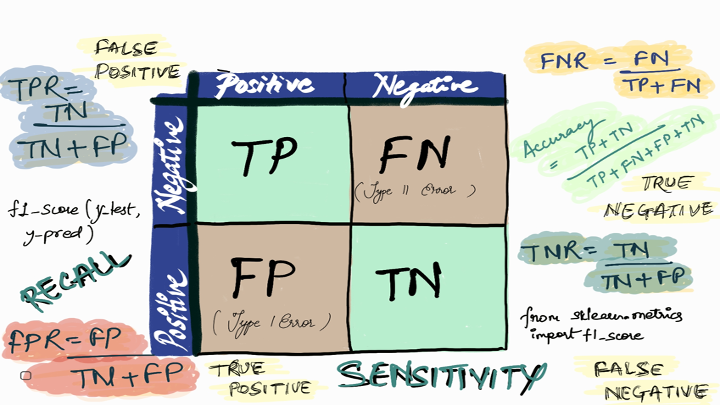Master LLMs with our FREE course in collaboration with Activeloop & Intel Disruptor Initiative. Join now!

## PublicationLatest

# Deep dive into Confusion Matrix

Last Updated on January 6, 2023 by Editorial Team

Last Updated on September 26, 2022 by Editorial Team

#### Author(s): Saurabh Saxena

Originally published on Towards AI the World’s Leading AI and Technology News and Media Company. If you are building an AI-related product or service, we invite you to consider becoming an AI sponsor. At Towards AI, we help scale AI and technology startups. Let us help you unleash your technology to the masses.

### Deep Dive Into Confusion Matrix

#### Precision (TPR), Recall (PPV), TNR, FPR, FNR, NPV, F1 Score, Accuracy, Balanced Accuracy, LR+, LR-

In the field of Data Science, model evaluation is the key component of the Training Lifecycle. There are many metrics to evaluate the classification model, but the Accuracy metric is often used. However, Accuracy might not give the correct depiction of the model due to class imbalance, and in such case, the Confusion Matrix is to be used for evaluation.

Confusion Matrix is pivotal to know, as many metrics are derived from it, be it precision, recall, F1-score, or Accuracy.

Let’s understand the metrics derived from the Confusion Matrix

True Positive (TP) is the number of correct predictions when the actual class is positive.

True Negative (TN) is the number of correct predictions when the actual class is negative.

False Positive (FP) is the number of incorrect predictions when the actual class is positive, also referred to as Type I Error.

False Negative (FN) is the number of incorrect predictions when the actual class is negative, also referred to as Type II Error.

`from sklearn.datasets import load_breast_cancerfrom sklearn.model_selection import train_test_split from sklearn.linear_model import LogisticRegressionfrom . import confusion_matrix`
`X, y = load_breast_cancer(return_X_y=True)X_train, X_test, y_train, y_test = train_test_split(X, y,                                                    test_size=0.33,                                                    random_state=42)lr= LogisticRegression()lr.fit(X_train,y_train) y_pred=lr.predict(X_test)`
`conf_mat = confusion_matrix(y_test, y_pred, plot=False)TP = conf_mat[0,0]TN = conf_mat[1,1]FP = conf_mat[1,0]FN = conf_mat[0,1]`
`print("TP: ", TP)print("TN: ", TN)print("FP: ", FP)print("FN: ", FN)`
`Output:TP:  63TN:  118FP:  3FN:  4`

True Positive Rate (TPR), Sensitivity, Recall: It is the probability of a person testing positive who has a disease. In other words, Recall is the proportion of examples of a particular class predicted by the model as belonging to that class.

`from sklearn.metrics import recall_scorerecall_score(y_test, y_pred)`
`Output:0.9752066115702479`

True Positive Rate (TPR), Specificity: It is the probability of a person testing negative who does not have a disease.

False Positive Rate (FPR), fall-out: It is the probability of a person testing positive who does not have a disease.

False Negative Rate (FNR), miss rate: It is the probability of a person testing negative who does have a disease.

`TNR = TN/(TN+FP)print("Specificity: ", TNR) FPR = FP/(TN+FP)print("FPR: ", FPR)FNR = FN/(TP+FN)print("FNR: ", FNR)`
`Output:Specificity:  0.9752066115702479FPR:  0.024793388429752067FNR:  0.05970149253731343`

Positive Predictive Value (PPV), Precision: It is the probability of a person having a disease who is tested positive. In other words, Precision is the proportion of correct predictions among all predictions.

`from sklearn.metrics import precision_scoreprecision_score(y_test, y_pred)`
`Output:0.9672131147540983`

Negative Predictive Value (NPV): It is the probability of a person not having a disease who is tested negative.

Positive likelihood ratio (LR+):

Negative likelihood ratio (LR-):

`TNR = TP/(TP+FN)NPV = TN/(TN+FN)print("NPV: ", NPV) LRp = TPR/FPRprint("LR+: ", LRp)LRn = FNR/TNRprint("LR-: ", LRn)`
`Output:NPV:  0.9672131147540983LR+:  37.92537313432836LR-:  0.06349206349206349`

Accuracy: Accuracy is the proportion of examples that were correctly classified. To be more precise, It is the ratio of correct prediction over the total number of cases.

`from sklearn.metrics import accuracy_scoreaccuracy_score(y_test, y_pred)`
`Output:0.9627659574468085`

Balanced Accuracy: It is the arithmetic mean of TPR and TNR. Balanced Accuracy finds its usage where data imbalance exists.

`from sklearn.metrics import balanced_accuracy_scorebalanced_accuracy_score(y_test, y_pred)`
`Output:0.9577525595164673`

F1 Score: It is the harmonic mean of precision and recall, so it’s an overall measure of the quality of a classifier’s predictions. It is usually the metric of choice for most people because it captures both precision and recall. It finds its way during Data Imbalance.

`from sklearn.metrics import f1_scoref1_score(y_test, y_pred)`
`Output:0.9711934156378601`

#### What is the difference between F1 and Balanced Accuracy?

F1 does not consider True Negative for evaluating the model, while Balanced Accuracy considers all four TP, TN, FP, and FN.

F1 is the composite metric where precision and recall are considered There are other composite metrics like precision-recall curve and ROC, and AUC, which are important to assess any classification model. To read more about these curves, please visit Precision-Recall and ROC Curve.

The below code is similar to the classification report of sklearn instead, it will give all metrics out of the confusion matrix for binary classification.

`report = binary_classification_report(y_test, y_pred)report`
`Output:{'TP': 118, 'TN': 63, 'FP': 4, 'FN': 3, 'TPR': 0.9752066115702479, 'Recall': 0.9752066115702479, 'Sensitivity': 0.9752066115702479, 'TNR': 0.9402985074626866, 'Specificity': 0.9402985074626866, 'FPR': 0.05970149253731343, 'FNR': 0.024793388429752067, 'PPV': 0.9672131147540983, 'Precision': 0.9672131147540983, 'Accuracy': 0.9627659574468085, 'Balaced Accuracy': 0.9577525595164673, 'F1 Score': 0.9711934156378601}`

Note: all the above codes mentioned in the blog are for binary classification,

In this blog, we understood the confusion matrix for binary classification. However, if you are interested in multiclass, please refer to Multi-class Model Evaluation with Confusion Matrix and Classification Report and if you are wondering about the “from . import confusion_matrix”, please refer to the Introduction to Confusion Matrix for the Python method.

References:

 sklearn metrics API. https://scikit-learn.org/stable/modules/classes.html#module-sklearn.metricsDeep dive into Confusion Matrix was originally published in Towards AI on Medium, where people are continuing the conversation by highlighting and responding to this story.

Join thousands of data leaders on the AI newsletter. It’s free, we don’t spam, and we never share your email address. Keep up to date with the latest work in AI. From research to projects and ideas. If you are building an AI startup, an AI-related product, or a service, we invite you to consider becoming a sponsor.

Published via Towards AI